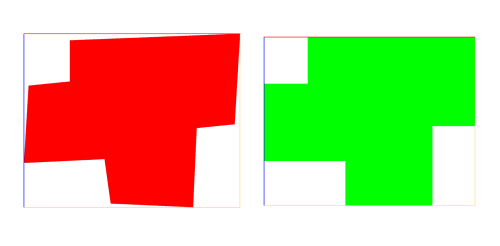# rectify

### Syntax

• rectify(angle)

### Parameters

1. anglefloat Maximum deviation in degrees from the right angle in range [0,45].

### Description

The rectify operation aligns edges to the scope such that they are parallel or perpendicular to each other if they initially deviate less than angle from that condition.

The algorithm aligns all edges such that their projection onto either the xy, yz or zx plane of the scope becomes parallel to the axes spanning that plane if the angle between the edge and the respective axis is less than  angle. From the planes xy, yz, zx the one that is most parallel to the shape is chosen as the projection plane.

Example: For a shape that is parallel to the xy plane of the scope, all edges with an angle less than angle to the x or y axis are aligned parallel to the x or y axis, respectively.

Limitations: The operation does not guarantee a good result for all cases, results should therefore be checked by the user. In some cases, the operation will remove holes or create self intersections. The operation is not suitable for initial shapes whose face normals point in very different directions, for example 3D solids.

### Example

Left: Initial shape; Right: Rectified shape``````@Range(min=0,max=45)
attr angle = 15

@StartRule
Lot -->
ShowOrig
ShowRect

ShowOrig -->
color(1, 0, 0)

ShowRect -->
t(0, 0, '-1.1)
rectify(angle)
color(0, 1, 0)``````